Check Class 3 CBSE maths measurements worksheets with answers. This has questions on the chapter 11  "Measurement of Length, Weight and Capacity". These worksheets follow the latest examination pattern and can be downloaded with answers in PDF format.  Answers and explanations for each question are available in the " buy courses" link given below.

Our paid course for Class 3 CBSE Maths  includes comprehensive chapterwise tests with answers covering all the chapters mentioned in the latest CBSE syllabus for Class 3. We provide explanations for each question at the end of the test. Our paid members can take each of the tests multiple times. CBSE study material for Class 3 at Olympiadtester strictly follows the NCERT, CBSE and KVS examination pattern.

To explore and purchase our courses please visit the  BUY COURSES section on our website.

1) What is the mass of A?1. 2 kg 175 g
2. 2 kg 900 g
3. 3 kg 625 g
4. 4 kg 350 g

2) Sheela has two kinds of square tiles.
Each small tile is 7 cm long.
Each big tile is 8 cm long.
She arranges all the small tiles in a row of 301 cm long, leaving no gaps in between.
She arranges all the big tiles in a row of 224 cm long, leaving no gaps in between.
How many more small tiles than big tiles does Sheela have?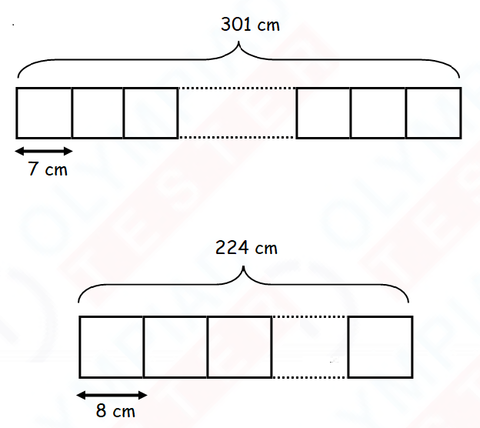1. 5
2. 11
3. 15
4. 26

3) 9 poles are arranged in a row with equal spacing in between them. If the distance between the first pole and the last pole is 72 m, what is the distance between each pole?

1. 8 m
2. 9 m
3. 63 m
4. 81 m

4) The School is 3 km from the Market.
The distance between the Library and the Market is 3 times as far as the school is from the library.
How far is the Library from the School?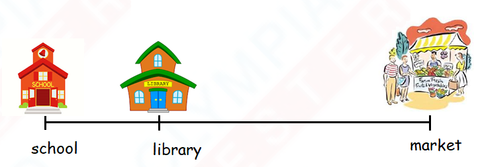5) A red ribbon is twice as long as a yellow ribbon.
The red ribbon is cut into 4 equal pieces.
If each piece is 17 cm long, find the length of the yellow ribbon.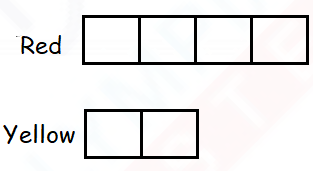6) The volume of water in the container is __________ mℓ.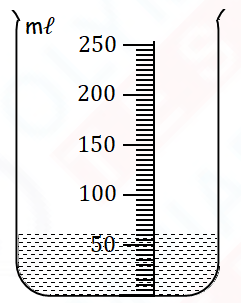1. 52
2. 54
3. 60
4. 70

7) 13 metres = ___________ centimetres.

1. 13
2. 130
3. 1300
4. 13 000

8) A can of orange juice is 300 ml. ​​​​​​​What is the minimum number of cans needed to fill up a container with a capacity of 2 litres?

Ans : ___________

9) Mr Seth walked 450 m to a bus stop.
Then he traveled by bus to the library.
The distance he traveled by bus was 2850 m longer than the distance he walked.
What was the total distance that Mr Seth traveled?

Ans: __________

10) The mass of a durian is 1740 g.
It is 780 g heavier than a pineapple.
What is the mass of the pineapple?

Ans: __________

11) The total mass of the watermelon and the papaya is __________ .1. 1 kg 500 g
2. 1 kg 100 g
3. 2 kg 100 g
4. 2 kg 600 g

12) Sue cut off 60 cm from a piece of ribbon which was 2 m 5 cm long. She then cut the remaining piece into 5 equal parts. What was the length of each small piece?

1. 29 cm
2. 38 cm
3. 145 cm
4. 725 cm

13) The mass of a box of apples is 3 kg. If the apples have a mass of 1 kg 270 g, what is the mass of the empty box?

1. 1 kg 730 g
2. 2 kg 270 g
3. 2 kg 730 g
4. 4 kg 270 g

14) The total length of 3 planks is 9 m 17 cm.
The 2nd plank is twice as long as the 1st plank.
The 3rd plank is twice as long as the 2nd plank.

What is the difference in length between the 1st and the 3rd plank?

Ans : ___________

15) 2 005 m = __________ km __________ m

Ans: __________

16) The mass of the bunch of grapes is __________________.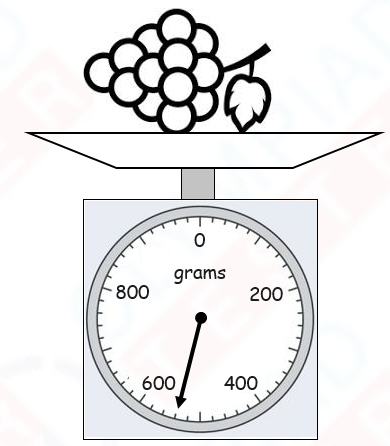1. 470 g
2. 520 g
3. 540 g
4. 570 g

17) The scale below shows the mass of a bag of rice. What is the mass of two such bags of rice?1. 5000 g
2. 2500 g
3. 10 kg
4. 5 kg

18) The length of rope A is twice that of rope B.
The length of rope B is thrice that of rope C.
Find the length of rope A if rope A is 80 cm longer than rope C.

19) What is the mass of the watermelon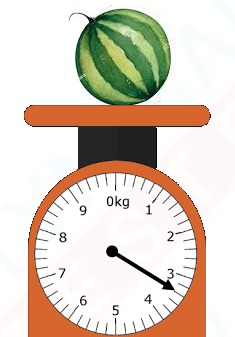20) Palm trees were planted along a pavement at an equal distance of 5 m apart from each other. ​​​​​​​What is the distance between the 1st tree and the 20th tree?

21) What is the volume of the water in the container?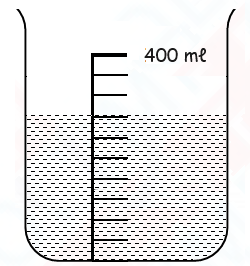22) Keya joined Cult club three years ago and her weight was 45 kg.
She lost 2 kg 500 g each year.
How heavy is she now?

23) Alok is 74 cm tall. His mother is 103 cm taller than him but 12 cm shorter than Alok's father.
​How tall is Alok's father?

24) If 1/2​  a tank can hold 32ℓ​​​​​​​ of water, how many litres of water can 3/4​  of the same tank hold?

25) The total mass of a table and a bicycle is 108kg. The table is twice the mass of the bicycle. What is the mass of the table?

1. 36 kg
2. 72 kg
3. 304 kg
4. 324 kg

26) The mass of a box with 8 marbles is 640 g. The mass of the same box with 12 marbles is 840 g. What is the mass of the box?

1. 50 g
2. 160 g
3. 200 g
4. 240 g

27) Pole A is 305 cm long. Pole B is twice as long as Pole A. Pole C is 350 cm shorter than Pole B. Find the difference in length between Pole A and Pole C.

1. 45 cm
2. 260 cm
3. 610 cm
4. 655 cm

28) The picture below shows the capacities of a pail and a vase.
What is the capacity of the pail?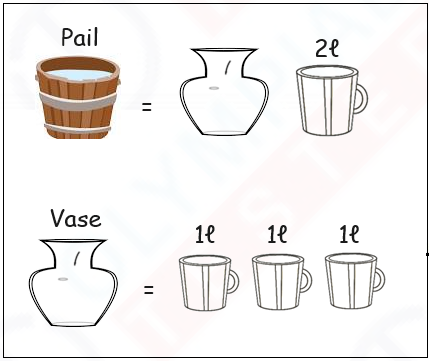1. 8ℓ​​​​​​​
2. 2ℓ​​​​​​​
3. 3ℓ​​​​​​​
4. 5ℓ​​​​​​​

29) May has two pieces of ribbon, A and B. Find the total length of both ribbons.1. 243cm
2. 322cm
3. 328cm
4. 407cm

30) A container has 800mℓ of water. When 900mℓ​​​​​​​ of water is added, 350 mℓ​​​​​​​ of water overflowed. What is the capacity of the container?